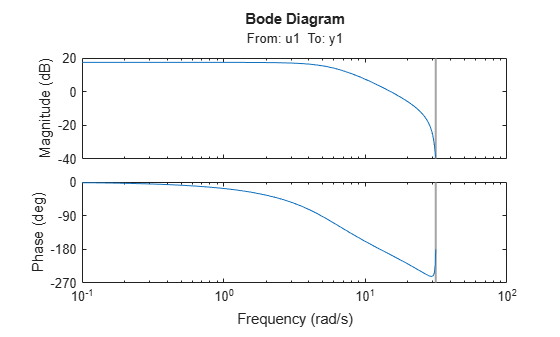# showConfidence

Display confidence regions on response plots for identified models

## Syntax

```showConfidence(plot_handle) showConfidence(plot_handle,sd) ```

## Description

`showConfidence(plot_handle)` displays the confidence region on the response plot, with handle `plot_handle`, for an identified model.

`showConfidence(plot_handle,sd)` displays the confidence region for `sd` standard deviations.

## Input Arguments

 `plot_handle` Response plot handle. `plot_handle` is the handle for the response plot of an identified model on which the confidence region is displayed. It is obtained as an output of one of the following plot commands: `bodeplot`, `stepplot`, `impulseplot`, `nyquistplot`, or `iopzplot`. `sd` Standard deviation of the confidence region. A common choice is 3 standard deviations, which gives 99.7% significance. Default: `getoptions(plot_handle,'ConfidenceRegionNumberSD')`

## Examples

collapse all

Show the confidence bounds on the bode plot of an identified ARX model.

Obtain identified model and plot its bode response.

```load iddata1 z1 sys = arx(z1, [2 2 1]); h = bodeplot(sys);````z1` is an `iddata` object that contains time domain system response data. `sys` is an `idpoly` model containing the identified polynomial model. `h` is the plot handle for the bode response plot of `sys`.

Show the confidence bounds for `sys`.

`showConfidence(h);`This plot depicts the confidence region for 1 standard deviation.

Show the confidence bounds on the bode plot of an identified ARX model.

Obtain identified model and plot its bode response.

```load iddata1 z1 sys = arx(z1, [2 2 1]); h = bodeplot(sys);````z1` is an `iddata` object that contains time domain system response data. `sys` is an `idpoly` model containing the identified polynomial model. `h` is the plot handle for the bode response plot of `sys`.

Show the confidence bounds for `sys` using 2 standard deviations.

```sd = 2; showConfidence(h,sd);````sd` specifies the number of standard deviations for the confidence region displayed on the plot.

## Alternatives

You can interactively turn on the confidence region display on a response plot. Right-click the response plot, and select Characteristics > Confidence Region.

## Version History

Introduced in R2012a